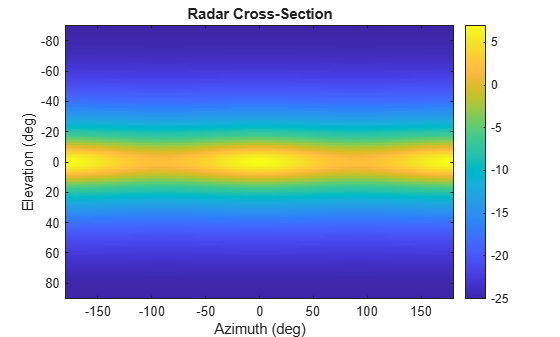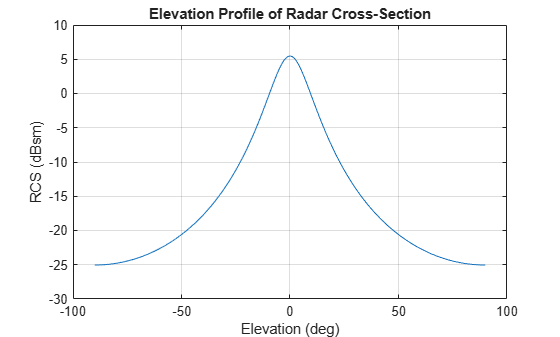# value

Radar cross-section at specified angle and frequency

## Syntax

``rcsval = value(rcssig,az,el,freq)``
``[rcsval,expval] = value(rcssig,az,el,freq)``

## Description

example

``` `rcsval = value(rcssig,az,el,freq)` returns the value, `rcsval`, of the radar cross-section (RCS) specified by the radar signature object, `rcssig`, computed at the specified azimuth `az`, elevation `el`, and frequency freq. If the specified azimuth and elevation is outside of the region in which the RCS signature is defined, the RCS value, `rcsval`, is returned as `-Inf` in dBsm.```
````[rcsval,expval] = value(rcssig,az,el,freq)` returns the expected values of the radar cross-section.```

## Input Arguments

expand all

Radar cross-section signature, specified as an `rcsSignature` object.

Azimuth angle, specified as scalar or length-M real-valued vector. Units are in degrees. The `az`, `el`, and `freq` arguments must have the same size. You can, however, specify one or two arguments as scalars, in which case the arguments are expanded to length-M.

Data Types: `double`

Elevation angle, specified as scalar or length-M real-valued vector. The `az`, `el`, and `freq` arguments must have the same size. You can, however, specify one or two arguments as scalars, in which case the arguments are expanded to length-M. Units are in degrees.

Data Types: `double`

RCS frequency, specified as a positive scalar or length-M vector with positive, real elements. The `az`, `el`, and `freq` arguments must have the same size. You can, however, specify one or two arguments as scalars, in which case the arguments are expanded to length-M vectors. Units are in Hertz.

Example: `100e6`

Data Types: `double`

## Output Arguments

expand all

Radar cross-section, returned as a scalar or real-valued length-M vector. Units are in dBsm.

Expected values of radar cross section, returned as a scalar or as a real-valued length-M vector. The dimensions of `expval` are the same as `rcsval`. Units are in dBsm.

Data Types: `double`

## Examples

expand all

Specify the radar cross-section (RCS) of a triaxial ellipsoid and plot RCS values along an azimuth cut.

Specify the lengths of the axes of the ellipsoid. Units are in meters.

```a = 0.15; b = 0.20; c = 0.95;```

Create an RCS array. Specify the range of azimuth and elevation angles over which RCS is defined. Then, use an analytical model to compute the radar cross-section of the ellipsoid. Create an image of the RCS.

```az = [-180:1:180]; el = [-90:1:90]; rcs = rcs_ellipsoid(a,b,c,az,el); rcsdb = 10*log10(rcs); imagesc(az,el,rcsdb) title('Radar Cross-Section') xlabel('Azimuth (deg)') ylabel('Elevation (deg)') colorbar```Create an `rcsSignature` object and plot an elevation cut at $3{0}^{\circ }$ azimuth.

```rcssig = rcsSignature('Pattern',rcsdb,'Azimuth',az,'Elevation',el,'Frequency',[300e6 300e6]); rcsdb1 = value(rcssig,30,el,300e6); plot(el,rcsdb1) grid title('Elevation Profile of Radar Cross-Section') xlabel('Elevation (deg)') ylabel('RCS (dBsm)')``````function rcs = rcs_ellipsoid(a,b,c,az,el) sinaz = sind(az); cosaz = cosd(az); sintheta = sind(90 - el); costheta = cosd(90 - el); denom = (a^2*(sintheta'.^2)*cosaz.^2 + b^2*(sintheta'.^2)*sinaz.^2 + c^2*(costheta'.^2)*ones(size(cosaz))).^2; rcs = (pi*a^2*b^2*c^2)./denom; end```

## Algorithms

The RCS signature, is first linearly interpolated at the specified azimuth, `az`, and elevation, `el`, view angles for the provided frequencies, `freq`. The interpolated signature is then used as an expected value of a probability distribution that generates a signature pattern value according to the RCS fluctuation model specified by the `FluctuationModel` property.. `az` and `el` are specified in degrees and are defined in the body frame of the pattern. `freq` is in hertz.

If `FluctuationModel` is `'Swerling0'`, the returned pattern value is a deterministic constant equal to the interpolated signature.

If `FluctuationModel` is `'Swerling1'`, the returned pattern value is a random variable distributed according to an exponential distribution with a mean value equal to the interpolated signature.

If `FluctuationModel` is `'Swerling3'`, the returned pattern value is a random variable distributed according to a chi-square distribution with four degrees of freedom and a mean value equal to the interpolated signature.

## Version History

Introduced in R2018b